Ch 1. Basics Multimedia Engineering Fluids MassDensity IdealGas Law Viscosity SurfaceTension VaporPressure
 Chapter 1. Basics 2. Fluid Statics 3. Kinematics 4. Laws (Integral) 5. Laws (Diff.) 6. Modeling/Similitude 7. Inviscid 8. Viscous 9. External Flow 10. Open-Channel Appendix Basic Math Units Basic Equations Water/Air Tables Sections Search eBooks Dynamics Fluids Math Mechanics Statics Thermodynamics Author(s): Chean Chin Ngo Kurt Gramoll ©Kurt GramollFLUID MECHANICS - THEORY

The ideal gas law will be introduced in this section. The conditions in which the ideal gas law is valid will be examined through the compressibility factor.

Ideal Gas Law

Table: Gas Constant for
Common Gases*
 Gas Constant, R (kJ/kg-K) Air 0.2870 Ammonia 0.4882 Carbon Dioxide 0.1889 Helium 2.0771 Hydrogen 4.1243 Nitrogen 0.2968 Oxygen 0.2598 R-12 0.06876 R-134a 0.08149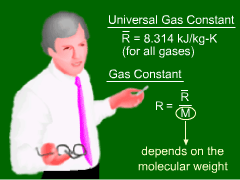Universal Gas Constant Versus
Gas Constant

The relationship between pressure and temperature for most gases can be approximated by the ideal gas law,

 pV = mRT

where p is the absolute pressure, V is the volume, m is the mass, T is the absolute temperature (units in Kelvin or Rankine) and R is the gas constant. Kelvin is related to Celsius by TK = TC + 273.15 and Rankine is related to Fahrenheit byTR = TF + 459.67.

This equation is also referred to as the perfect gas law or the equation of state for an ideal gas. The gas constant R for some common gases is given in the table. Note that the density ρ is given by m/V, hence the ideal gas law can be written in terms of the density as

p = ρRT

The ideal gas law can also be written in per mole basis as follows: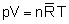where n is the number of moles and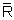is the universal gas constant. The number of moles is given by n = m/M where M is the molecular weight of the gas. The universal gas constantis 8.314 kJ/kmol-K for all gases, and it is related to the gas constant by: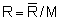Compressibility Factor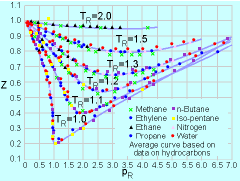Compressibility Chart for
Various Gases**

Table: Critical Pressure and
Temperature for Various Gases*
 CriticalTemp.Tc (K) CriticalPressurepc (MPa) Air 133.0 3.77 Ammonia 405.5 11.3 CarbonDioxide 304.1 7.38 Helium 5.19 0.227 Hydrogen 33.2 1.30 Nitrogen 126.2 3.39 Oxygen 154.6 5.04 R-12 385.0 4.14 R-134a 374.2 4.06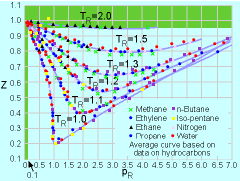Validity of the Ideal Gas Law**

Having introduced the ideal gas law, the next important question is: Under what conditions will the ideal gas law be a good approximation for real gases? To address this issue, the compressibility factor Z is introduced, and it is defined as:

Z = pV/mRT

Obviously, when Z approaches unity, then the ideal gas law holds.

The compressibility factor for various gases is plotted as a function of the reduced pressure (pR) and reduced temperature (TR) in the compressibility chart as shown in the figure. The reduced pressure and temperature are defined as follows:

pR = p/pc
TR = T/Tc

where pc and Tc are the critical pressure and critical temperature, respectively. The critical temperature is the maximum temperature that liquid and vapor phases can coexist in equilibrium. The corresponding pressure is the critical pressure. Values of critical pressure and temperature for some common gases are summarized in the table.

From the compressibility chart, it is observed that Z approaches unity when pR < 0.1 or TR > 2. Hence, these are the conditions when the behavior of real gases can best be approximated using the ideal gas law.

* Source: Sonntag, R. E. and Borgnakke, C., "Introduction to Engineering Thermodynamics," John Wiley, Inc., New York, 2001.

** Reference: Su, G. J., "Modified Law of Corresponding States," Ind. Eng. Chem. (international ed.), Vol. 38, pp. 803, 1946.

Practice Homework and Test problems now available in the 'Eng Fluids' mobile app
Includes over 250 problems with complete detailed solutions.
Available now at the Google Play Store and Apple App Store.